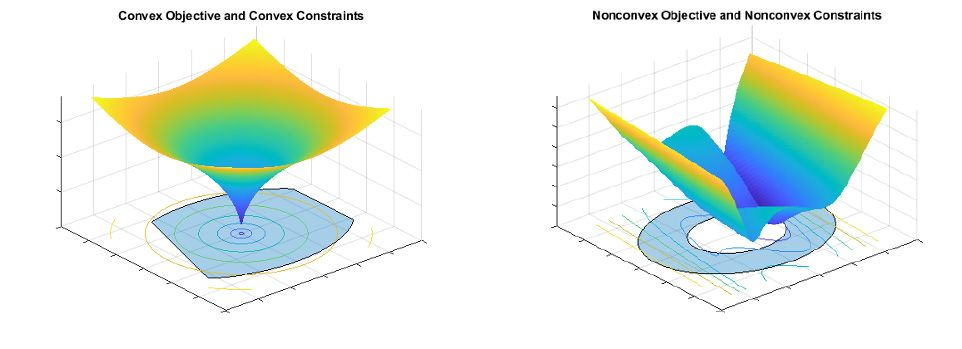Convex Optimization

Minimize convex functions over convex regions

Convex optimization is the process of minimizing a convex objective function subject to convex constraints or, equivalently, maximizing a concave objective function subject to convex constraints. Points satisfying local optimality conditions can be found efficiently for many convex optimization problems. Because a point that is a local optimum is also a global optimum, it is sufficient to find a local optimum to solve the problem. Convex approximations of nonconvex problems provide bounds on the optimal objective value and approximate solutions.

The figures below show examples of convex and nonconvex optimization problems.Applications of convex optimization are found in finance and engineering, including portfolio optimization, design optimization, parameter estimation, signal processing, and optimal control. For example, the problem of selecting a portfolio of stocks to maximize return subject to upper bounds on risk and tracking error against a benchmark portfolio can be formulated as a convex optimization problem.

Convex optimization is the mathematical problem of finding a vector $$x$$ that minimizes the function:

$$min_{x} f(x)$$

subject to:

$$g_i (x)≤0$$ (nonlinear inequality constraints)

$$Ax ≤b$$ (linear inequality constraints)

$$A_{eq} x=b_{eq}$$ (linear equality constraints)

$$lb ≤x ≤ub$$ (bound constraints)

where $$g_i,i = 1,…,m$$ are convex functions.

Linear programs (LP) and convex quadratic programs (QP) are convex optimization problems. Conic optimization problems, where the inequality constraints are convex cones, are also convex optimization problems. Problems with linear or convex quadratic objectives and linear and convex quadratic constraints (QCQP) can be represented as second-order cone programs (SOCP), which enables solving by efficient convex optimization methods.

Interior point algorithms are commonly used to solve convex optimization problems and can be written in MATLAB® using matrix operations and the Cholesky factorization or the block LDL’ factorization. Optimization Toolbox™ has implementations of interior point algorithms for linear programs, quadratic programs, nonlinear programs, and second-order cone programs that are suitable for large-scale problems.